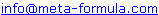# Metastock Formulas - K Click here to go back to Metastock Formula Index

 IMPORTANT: These formulas aren't my complete collection. For my complete collection of instantly usable, profitable and powerful MetaStock formulas Click Here

Would You Like To Use MetaStock To Its Full Potential? Discover the Amazingly Simple Secret to Master Metastock Step-By-Step - click here

A | A 1 | B | B 1 | C | C 1 | D | D 1 | E | F | G | H | I | J | K | L | M | M 1 | N | O | P | R | S | S 1 | T | U | V | W | Z |

 KA Money Flow System Karnish Bollinger Band Histogram Trading System Kurtosis Indicator Kauffman's Adaptive RSI Krausz's Gann Swing HiLow Activator Keltner Channels

## KA Money Flow System

by Karim Anwar

This system is based on Money Flow Index . It is a developing system and will like input from other readers to improve it> Thanks for any suggestions to improve it.

It tries to buy when MFI is starting to rally from an oversold state and sells short when MFI starts falling from overbought state.

Can any body help to form an exploration to find stocks for whom this system will work best ?

EnterLong: Cross(MFI(23), (LLV(MFI(23),23) + opt1)) AND MFI(23)<50

closeLong: MFI(23)=LLV(MFI(23),23)

longOptimisationFullDetails: OPT 1 = Min. 5 Max. 20 Step: 1

enterShort: Cross( (HHV(MFI(23),23) - opt1), MFI(23))AND MFI(23)>50

closeShort: MFI(23)=HHV(MFI(23),23)

ShortOptimisationFullDetails: OPT 1 = Min. 5 Max. 20 Step: 1

(Go Top)

## Karnish Bollinger Band Histogram Trading System

{EnterLong and Close Short}
BBHistogram:= (CLOSE + 2*Std(CLOSE,20) -
Mov(CLOSE,20,SIMPLE)) / (4*(Std(CLOSE,20)))
* 100;
Cross(0,BBHistogram)

{Enter Short and Close Long}
BBHistogram:= (CLOSE + 2*Std(CLOSE,20) -
Mov(CLOSE,20,SIMPLE)) / (4*(Std(CLOSE,20)))
* 100;
Cross(BBHistogram,100)

Here's a "freebie".
BB Histogram:
((C+2*Std(C,20)-Mov(C,20,S))/(4*(Std(C,20)))*100)

Sell the opening days after the BB Histogram penetrates 100 and buy when it penetrates zero. Add to positions when the BB Histo leaves "above 100" or "below zero" and then "repenetrates" the trigger levels.

I believe this approach has recorded 11 straight S&P winners, with 700+ points. "But Steve, this system must not be working any more because it is losing the last trade you put on". Right!

My only disclaimer is that I guarantee that I will sell software, charting services and anything else that I can think of to make a "buck" in 2000. In the meantime, suck all the free stuff from me you can copy. And most of all, please note, the biggest antagonists on the list provide absolutely "zero" when it comes to helping you trade. Seek the answers from "within" (with some shortcutting help from people that are willing to share).

(Go Top)

MetaStock formula derived from calculations in Trading Systems and Methods, Third Edition, by Perry J. Kaufman.
This formula adapts the standard RSI to a smoothing constant.

Period := Input("Period",1,10000,20);

sc := Abs(RSI(Period)/100 - .5)*2;

If(Cum(1) <= Period, CLOSE,
PREV + sc*(CLOSE - PREV))

(Go Top)

## Krausz's Gann Swing HiLow Activator

I was only able to implement Krausz's Gann Swing HiLow Activator in Metastock,
because it's simply the average of the last three bars High (stop for short position or
long entry) or Low (stop for long position or short entry) plotted one period forward:

Ref(Mov(L,3,S),-1) or Ref(Mov(H,3,S),-1)

(Go Top)

## Kurtosis Indicator

The Kurtosis is a market sentiment indicator. The MetaStock formula for the Kurtosis is as follows:

Mov(Mov(Mo(3)-Ref(Mo(3),-1),66,E),3,S)

The Kurtosis is constructed from three different parts. The Kurtosis, the Fast Kurtosis(FK), and the Fast/Slow Kurtosis(FSK).

The Kurtosis(K) portion of this calculation is mo(3)-ref(mo(3),-1). Which is today's Kurtosis - yesterday's Kurtosis.

The Fast Kurtosis(FK) is mov(K,66,E) mov(mo(3)-ref(mo(3),-1,66,E). Which is the Kurtosis smoothed with a 66 period exponential moving average.

The Fast/Slow Kurtosis(FSK) is the complete formula mov(mov(mo(3)-ref(mo(3),-1),66,E),3,S). Which is the FK smoothed with 3 period simple moving average.

**You may want to experiment with different time periods to optimize the results. For example, to calculate a 4 period Kurtosis, a 50 period FK and a 10 period FSK, use the following formula:

mov(mov(mo(4)-ref(mo(4),-1),50,E),10,S)

(Go Top)

## Keltner Channels

Keltner Channels are explained in the book The New Commodity Trading System and Methods by Perry Kaufman and were first introduced in the book How To Make Money in Commodities, by Chester W. Keltner. The syntax for the formulas in MetaStock are:

Formula #1
 Name: The 10-Day Moving Average Formula: MOV( (H+L+C)/3, 10, Simple )
Formula #2
 Name: Upper Keltner Band Formula: MOV((H+L+C)/3,10,S) + MOV((H-L),10,S)
Formula #3
 Name: Lower Keltner Band Formula: MOV((H+L+C)/3,10,S) - MOV((H-L),10,S)

** All three formulas must be plotted.

In the System Tester, a BUY or a COVER SHORT Signal would occur when

C > Upper Keltner Band

and a SELL or a SELL SHORT Signal would occur when

C < Lower Keltner Band

** Thus, the system is always either long or short.

For interpretation refer to either of the two books listed above.

(Go Top)

If you have Metastock formulas you would like to share,
Please email toWe look forward to hearing from you!